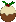The general Runge-Kutta algorithm is one of a few algorithms for solving first order ordinary differential equations. Below is a specific implementation for solving equations of motion and other second order ordinary differential equations (ODEs) for Physics simulations, amongst other things.

To see it at work, there’s a demo below, or check out my elastic cursor trailer for a more complex version.

The basic working behind the RK4 is to take snapshots of a particle’s motion as it travels. To do this, it takes in the “state” of the system – in this case, the particle’s position and velocity – and the time step from the last iteration, and uses that along with the acceleration function you provide to compute where the particle should be and how fast it should be travelling after that time step. This is then the new state of the system. You can read more about the Runge-Kutta algorithm on Wikipedia.

The main RK4 function:

  1 2 3 4 5 6 7 8 9 10 11 12 13 14 15 16 17 18 19 20 21 22 23 24 25 26 27 28  // Converted from Python version: http://doswa.com/2009/01/02/fourth-order-runge-kutta-numerical-integration.html function rk4(x, v, a, dt) { // Returns final (position, velocity) array after time dt has passed. // x: initial position // v: initial velocity // a: acceleration function a(x,v,dt) (must be callable) // dt: timestep var x1 = x; var v1 = v; var a1 = a(x1, v1, 0); var x2 = x + 0.5*v1*dt; var v2 = v + 0.5*a1*dt; var a2 = a(x2, v2, dt/2); var x3 = x + 0.5*v2*dt; var v3 = v + 0.5*a2*dt; var a3 = a(x3, v3, dt/2); var x4 = x + v3*dt; var v4 = v + a3*dt; var a4 = a(x4, v4, dt); var xf = x + (dt/6)*(v1 + 2*v2 + 2*v3 + v4); var vf = v + (dt/6)*(a1 + 2*a2 + 2*a3 + a4); return [xf, vf]; }

Here’s a simple little simple harmonic motion (SHM) demo to show you what it does and how to use it:

Start DemoJavaScript:

  1 2 3 4 5 6 7 8 9 10 11 12 13 14 15 16 17 18 19 20 21  window.startDemo=function() { window.state = [- document.getElementById("b").offsetWidth / 2 - 10, 0]; window.dt = 1/50; // Length of each frame in seconds window.interval = setInterval(function() { var b = document.getElementById("b"); // Box var p = document.getElementById("p"); // Particle window.state = rk4(window.state, window.state, (function(x, v, dt) { // This is the acceleration function // This particular one models a spring with a 1kg mass var stiffness=400,damping=0.25; return -stiffness*x-damping*v; }), window.dt); p.style.left = b.offsetWidth/2 + (window.state || 0) + "px"; // Stop iterating when it comes to rest at the centre if (Math.round(window.state)==0 && Math.round(window.state)==0) clearInterval(window.interval); }, window.dt*1000); }

HTML:

 1 2 3  
*